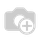# [Odoo 10] How to get the static files into a model file ?

##### I use the following code to search the image but it does not work.
`import osimport base64class Users(object):    def __init__(self, login, image):        self.login = login        self.image = imageimg = os.path.join('name_of_the_module', 'static','src', 'img', login+'.jpg')values = Users("test.test", base64.b64encode(img))self.env['res.users'].sudo().create(values)`
##### Robin Hède.I finally found a solution to my problem.

Here is the code:

`from odoo import modules, tools`
`login = "test.test"`
`class Users(object):`
`    def __init__(self, login, image):`
`        self.login = login`
`        self.image = image`
`image_path = modules.module.get_resource_path(`
`    'name_of_the_module',`
`    'static/img',`
`    login+'.jpg'`
`)`
`if image_path:`
`    image = tools.image_resize_image_big(`
`        open(`
`            image_path,`
`            'rb'`
`                ).read().encode('base64')`
`    )`
`else:`
`    image = ''`
`values = Users(login, image)`
`self.env['res.users'].sudo().create(values)Best regards,Robin Hède.`